# Lesson 44 Optimization Calculus Santowski 962021 Calculus Santowski

• Slides: 29Lesson 44 – Optimization Calculus - Santowski 9/6/2021 Calculus - Santowski 1Lesson Objectives • 1. Solve optimization problems using a variety of calculus and non-calculus based strategies • 2. Solve optimization problems with and without the use of the TI 84 GDC • 3. Work with problems set in mathematical contexts or in the context of a real world problem 9/6/2021 Calculus - Santowski 2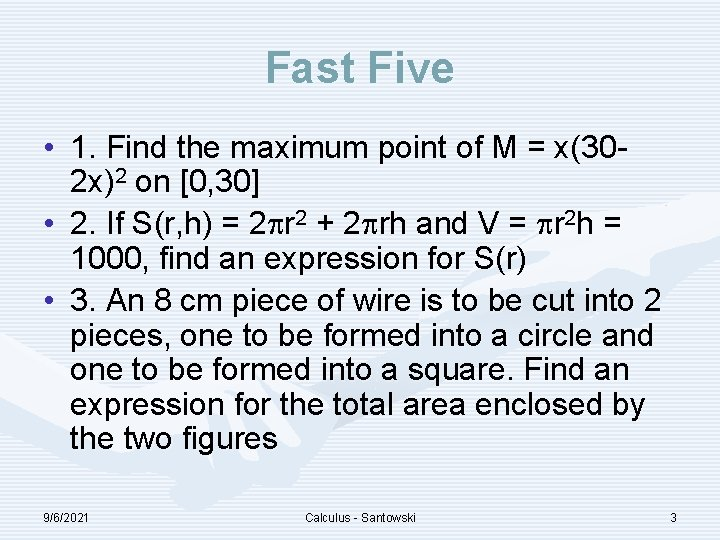Fast Five • 1. Find the maximum point of M = x(302 x)2 on [0, 30] • 2. If S(r, h) = 2 r 2 + 2 rh and V = r 2 h = 1000, find an expression for S(r) • 3. An 8 cm piece of wire is to be cut into 2 pieces, one to be formed into a circle and one to be formed into a square. Find an expression for the total area enclosed by the two figures 9/6/2021 Calculus - Santowski 3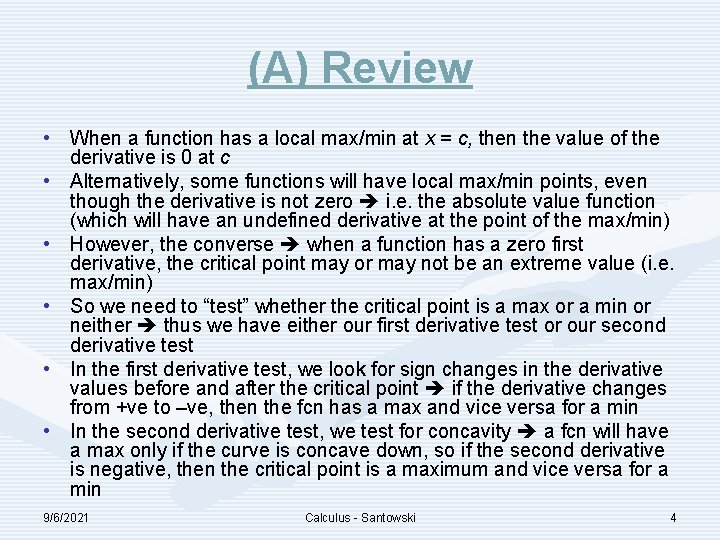(A) Review • When a function has a local max/min at x = c, then the value of the derivative is 0 at c • Alternatively, some functions will have local max/min points, even though the derivative is not zero i. e. the absolute value function (which will have an undefined derivative at the point of the max/min) • However, the converse when a function has a zero first derivative, the critical point may or may not be an extreme value (i. e. max/min) • So we need to “test” whether the critical point is a max or a min or neither thus we have either our first derivative test or our second derivative test • In the first derivative test, we look for sign changes in the derivative values before and after the critical point if the derivative changes from +ve to –ve, then the fcn has a max and vice versa for a min • In the second derivative test, we test for concavity a fcn will have a max only if the curve is concave down, so if the second derivative is negative, then the critical point is a maximum and vice versa for a min 9/6/2021 Calculus - Santowski 4Intro to Our Examples • We will work through several examples together, wherein we outline the key steps involved in the process of determining max/min values in application based problems 9/6/2021 Calculus - Santowski 5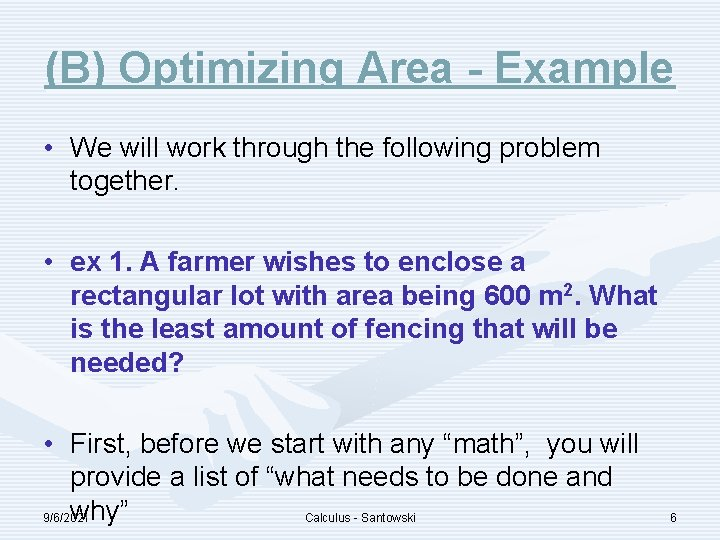(B) Optimizing Area - Example • We will work through the following problem together. • ex 1. A farmer wishes to enclose a rectangular lot with area being 600 m 2. What is the least amount of fencing that will be needed? • First, before we start with any “math”, you will provide a list of “what needs to be done and why” 9/6/2021 Calculus - Santowski 6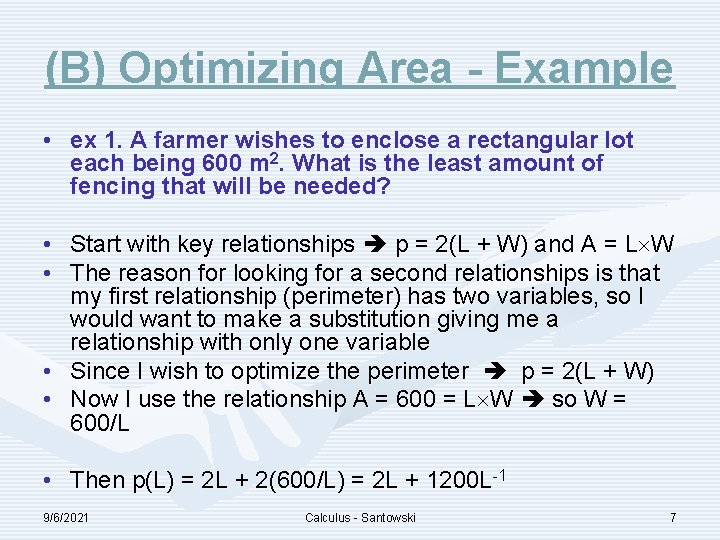(B) Optimizing Area - Example • ex 1. A farmer wishes to enclose a rectangular lot each being 600 m 2. What is the least amount of fencing that will be needed? • Start with key relationships p = 2(L + W) and A = L W • The reason for looking for a second relationships is that my first relationship (perimeter) has two variables, so I would want to make a substitution giving me a relationship with only one variable • Since I wish to optimize the perimeter p = 2(L + W) • Now I use the relationship A = 600 = L W so W = 600/L • Then p(L) = 2 L + 2(600/L) = 2 L + 1200 L-1 9/6/2021 Calculus - Santowski 7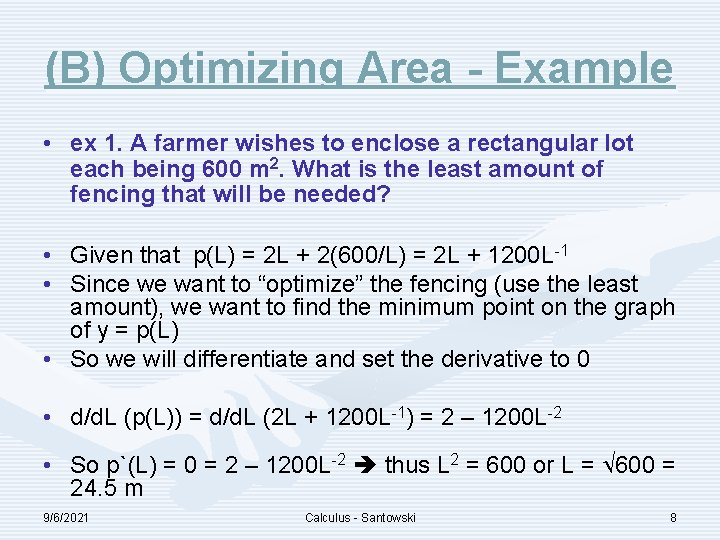(B) Optimizing Area - Example • ex 1. A farmer wishes to enclose a rectangular lot each being 600 m 2. What is the least amount of fencing that will be needed? • Given that p(L) = 2 L + 2(600/L) = 2 L + 1200 L-1 • Since we want to “optimize” the fencing (use the least amount), we want to find the minimum point on the graph of y = p(L) • So we will differentiate and set the derivative to 0 • d/d. L (p(L)) = d/d. L (2 L + 1200 L-1) = 2 – 1200 L-2 • So p`(L) = 0 = 2 – 1200 L-2 thus L 2 = 600 or L = 600 = 24. 5 m 9/6/2021 Calculus - Santowski 8(B) Optimizing Area - Example • Now, we need to verify that the critical point that we generated (when L = 24. 5 m) is actually a minimum point. • We can use the first derivative test and we can substitute L = 20 m and L = 25 m into the derivative equation • p`(20) = 2 – 1200(20)-2 = 2 – 3 = -1 • p`(25) = 2 – 1200(25)-2 = 2 – 1. 92 = +0. 08 • So our derivative changes from –ve to +ve as L increases, so our function must have a minimum point at 24. 5 m • Alternatively, we can use the second derivative test • p``(L) = -1200 x -2 L-3 = 2400/L 3 • Then p``(24. 5) = 2400/(24. 53) = +0. 163 which means that the second derivative is positive at L = 24. 5 m meaning our function is concave up which means that our critical value at L = 24. 5 must correspond to a minimum 9/6/2021 Calculus - Santowski 9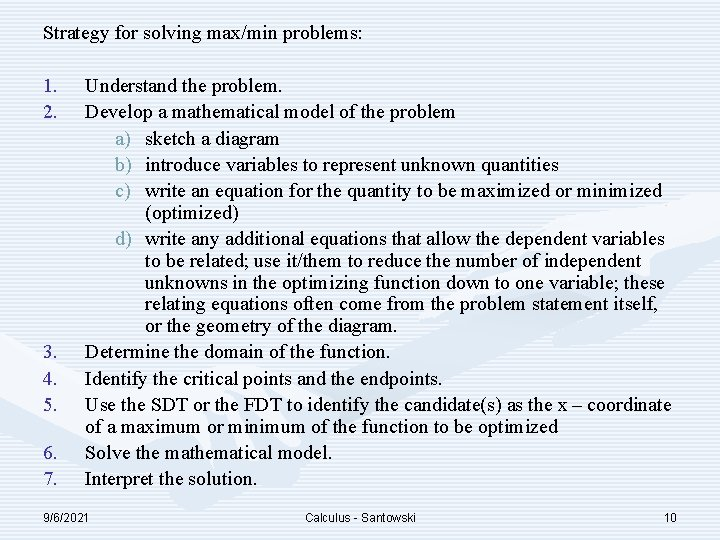Strategy for solving max/min problems: 1. 2. 3. 4. 5. 6. 7. Understand the problem. Develop a mathematical model of the problem a) sketch a diagram b) introduce variables to represent unknown quantities c) write an equation for the quantity to be maximized or minimized (optimized) d) write any additional equations that allow the dependent variables to be related; use it/them to reduce the number of independent unknowns in the optimizing function down to one variable; these relating equations often come from the problem statement itself, or the geometry of the diagram. Determine the domain of the function. Identify the critical points and the endpoints. Use the SDT or the FDT to identify the candidate(s) as the x – coordinate of a maximum or minimum of the function to be optimized Solve the mathematical model. Interpret the solution. 9/6/2021 Calculus - Santowski 10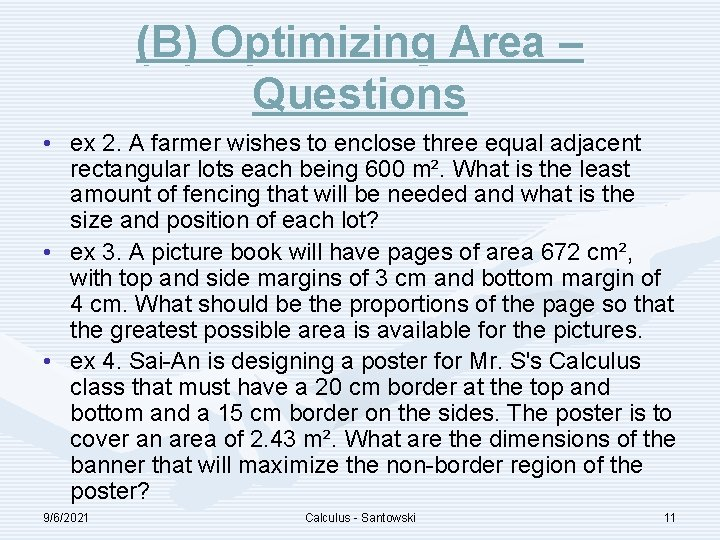(B) Optimizing Area – Questions • ex 2. A farmer wishes to enclose three equal adjacent rectangular lots each being 600 m². What is the least amount of fencing that will be needed and what is the size and position of each lot? • ex 3. A picture book will have pages of area 672 cm², with top and side margins of 3 cm and bottom margin of 4 cm. What should be the proportions of the page so that the greatest possible area is available for the pictures. • ex 4. Sai-An is designing a poster for Mr. S's Calculus class that must have a 20 cm border at the top and bottom and a 15 cm border on the sides. The poster is to cover an area of 2. 43 m². What are the dimensions of the banner that will maximize the non-border region of the poster? 9/6/2021 Calculus - Santowski 11(B) Optimizing Area – Questions 9/6/2021 Calculus - Santowski 12(B) Optimizing Area – Questions 9/6/2021 Calculus - Santowski 13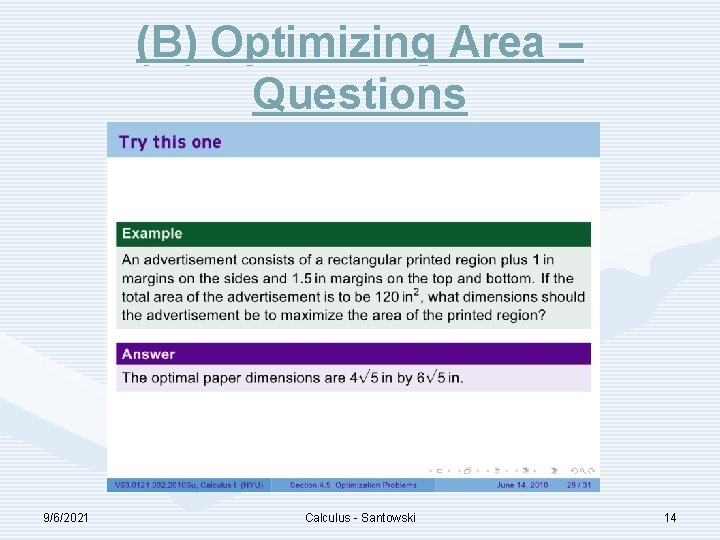(B) Optimizing Area – Questions 9/6/2021 Calculus - Santowski 14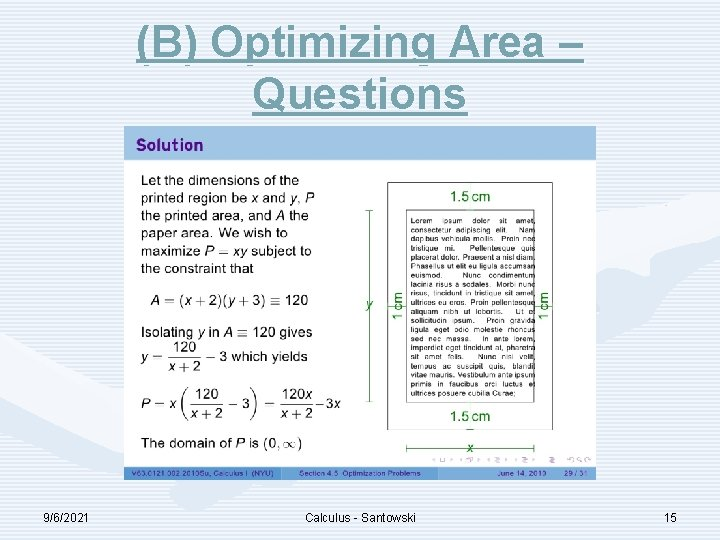(B) Optimizing Area – Questions 9/6/2021 Calculus - Santowski 15(B) Optimizing Area – Questions 9/6/2021 Calculus - Santowski 16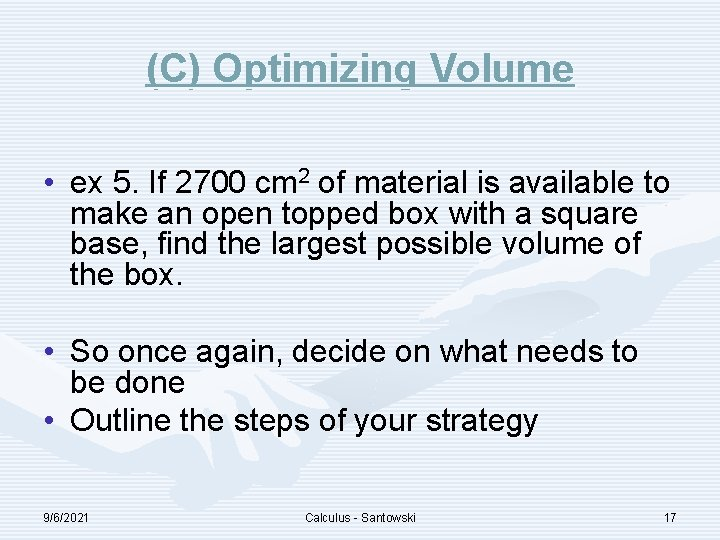(C) Optimizing Volume • ex 5. If 2700 cm 2 of material is available to make an open topped box with a square base, find the largest possible volume of the box. • So once again, decide on what needs to be done • Outline the steps of your strategy 9/6/2021 Calculus - Santowski 17(C) Optimizing Volume • ex 5. If 2700 cm 2 of material is available to make an open topped box with a square base, find the largest possible volume of the box. • Start with what you want to “optimize” in this case the volume so we need a volume formula V = L W H • We know that the base is a square (so L = W) so we can modify our formula as V = L L H = L 2 H • But we are also given info. about the surface area (the material available to us), so we will work with a second relationship • The surface area of a square based, on top box would be SA = L L + 4 L H • Thus 2700 cm 2 = L 2 + LH then H = (2700 – L 2)/L • So now we can finalize our formula, before differentiating as: • V(L) = L 2 (2700 – L 2)/L = L (2700 – L 2) = 2700 L – L 3 • But now let’s consider the domain of our function (0, √ 2700) 9/6/2021 Calculus - Santowski 18(D) Optimizing With Three Dimensional Geometric Shapes • Ex 6. A can is made to hold 1 L of oil. Find the dimensions that will minimize the cost of the metal needed to manufacture the can. • So once again, decide on what needs to be done • Outline the steps of your strategy 9/6/2021 Calculus - Santowski 19(D) Optimizing With Three Dimensional Geometric Shapes • Ex 6. A can is made to hold 1 L of oil. Find the dimensions that will minimize the cost of the metal needed to manufacture the can. • we wish to minimize the surface area, so the surface area formula for cylinders is the place to start • SA(r, h) = 2 r 2 + 2 rh = 2 r(r + h) • Now again, we have 2 variables, so we need to express one in terms of the other • The volume of a cylinder is V = r 2 h = 1000 cm 3 = 1 L • So 1000/ r 2 = h • Therefore SA(r) = 2 r(r + 1000/ r 2 ) = 2 r 2 + 2000 r-1 • And the domain would be? Well SA > 0, so 2 r 2 + 2000 r 1 > 0 9/6/2021 Calculus - Santowski 20(D) Optimizing With Three Dimensional Geometric Shapes • Ex 6. A cylindrical can is made to hold 1 L of oil. Find the dimensions that will minimize the cost of the metal needed to manufacture the can. • Now differentiate to find the critical points on the SA function • SA`(r) = 4 r – 2000 r-2 = 0 r 3 = 500/ r = 5. 42 cm h = 10. 8 cm • Now let’s run through the first derivative test to verify a minimum SA • SA`(5) = 4 (5) – 2000(5)-2 = -17. 2 • SA`(6) = 4 (6) – 2000(6)-2 = 19. 8 • So the first derivative changes from –ve to +ve, meaning that the value r = 5. 42 cm does represent a minimum 9/6/2021 Calculus - Santowski 21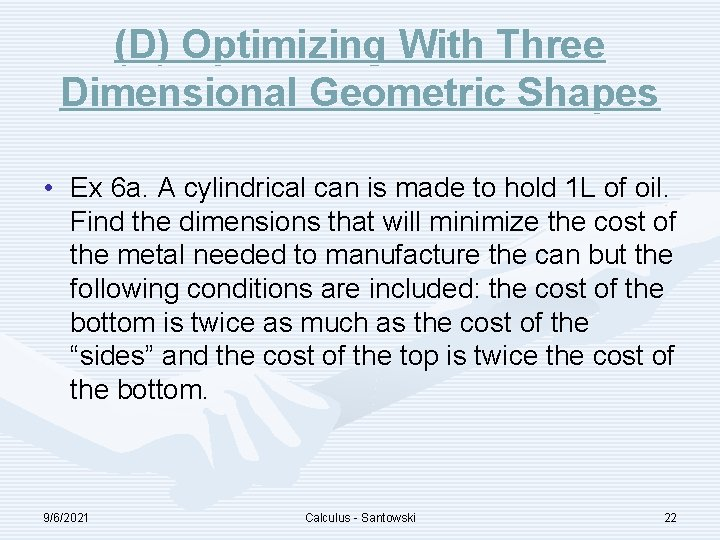(D) Optimizing With Three Dimensional Geometric Shapes • Ex 6 a. A cylindrical can is made to hold 1 L of oil. Find the dimensions that will minimize the cost of the metal needed to manufacture the can but the following conditions are included: the cost of the bottom is twice as much as the cost of the “sides” and the cost of the top is twice the cost of the bottom. 9/6/2021 Calculus - Santowski 22(D) Optimizing With Three Dimensional Geometric Shapes • ex 7. A cylinder is inscribed in a right circular cone of altitude 12 cm and radius of base is 4 cm. Find the dimensions of the circular cylinder that will make the total surface area a maximum. • ex 8. Find the dimensions of the circular cylinder of greatest possible volume that could be inscribed in a sphere of radius 4 cm. 9/6/2021 Calculus - Santowski 23(D) Optimizing With Three Dimensional Geometric Shapes • Ex 9. Naomi is designing a cylindrical can which will hold 280 m. L of juice. The metal for the sides costs \$0. 75/m 2 while the metal for the top and bottom costs \$1. 40/m 2. Find the dimensions that will minimize the cost of the materials. • Ex 10. A tank with a rectangular base and rectangular sides is open at the top. It is to be constructed so that its width is 4 meters and its volume is 36 cubic meters. If building the tank costs \$10/sq. m. for the base and \$5/sq. m. for the sides, what is the cost of the least expensive tank, and what are its dimensions? 9/6/2021 Calculus - Santowski 24(E) Optimizing Revenue • ex 11. The local orchestra wants to raise the price for season tickets for next year. Currently, there are 760 season ticket holders who paid \$200 each. It is estimated that for every \$10 increase in price, 20 subscribers will not buy a season ticket. What season ticket price will maximize revenue? • ex 12. Steve is driving a truck on the highway which burns fuel at the rate of (0. 003 x + 3/x) litres per kilometer when its speed is x km/h. Fuel costs \$0. 65/L and Steve is paid \$16/hr. What steady speed will minimize the operating costs for a 400 km Calculus trip on a highway? 9/6/2021 - Santowski 25(F) Further Examples • ex 13. A north-south highway intersects an eastwest highway at point P. A vehicle passes point P at 1: 00 pm traveling east at a constant speed of 60 km/h. At the same instant, another vehicle is 5 km north of P, traveling south at 80 km/h. Find the time when the two vehicles are closest to each other and the distance between them at that time. • Show to come up with a distance equation of d 2 = (5 - 80 t)2 + (60 t)2 9/6/2021 Calculus - Santowski 26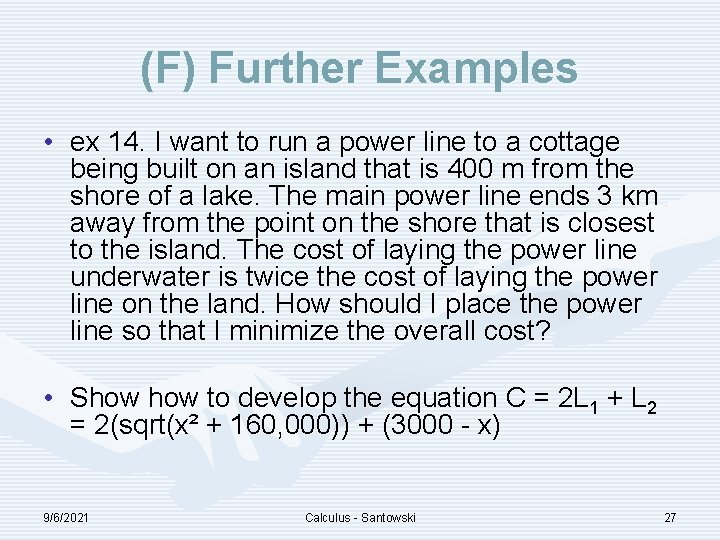(F) Further Examples • ex 14. I want to run a power line to a cottage being built on an island that is 400 m from the shore of a lake. The main power line ends 3 km away from the point on the shore that is closest to the island. The cost of laying the power line underwater is twice the cost of laying the power line on the land. How should I place the power line so that I minimize the overall cost? • Show to develop the equation C = 2 L 1 + L 2 = 2(sqrt(x² + 160, 000)) + (3000 - x) 9/6/2021 Calculus - Santowski 27(F) Further Examples • An offshore well is located in the ocean at a point W which is six miles from the closest shore point A on a straight shoreline. The oil is to be piped to a shore point B that is eight miles from A by piping it on a straight line under water from W to some shore point P between A and B and then on to B via a pipe along the shoreline. If the cost of laying pipe is \$100, 000 per mile under water and \$75, 000 per mile over land, how far from A should the point P be located to minimize the cost of laying the pipe? What will the cost be? 9/6/2021 Calculus - Santowski 28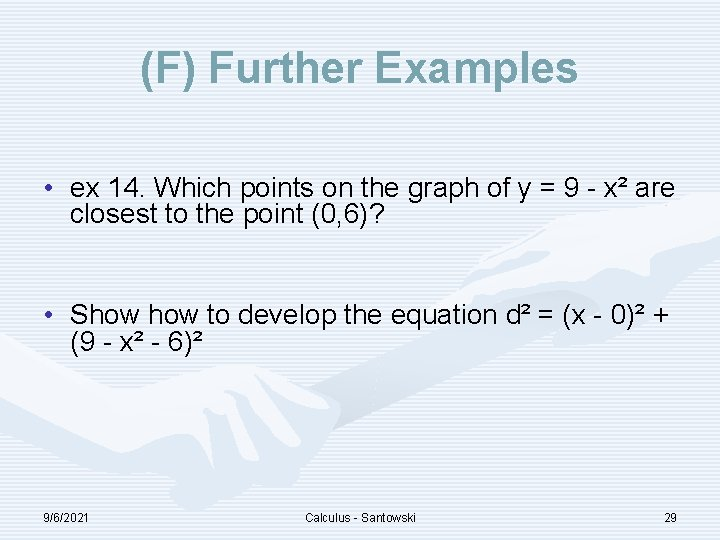(F) Further Examples • ex 14. Which points on the graph of y = 9 - x² are closest to the point (0, 6)? • Show to develop the equation d² = (x - 0)² + (9 - x² - 6)² 9/6/2021 Calculus - Santowski 29